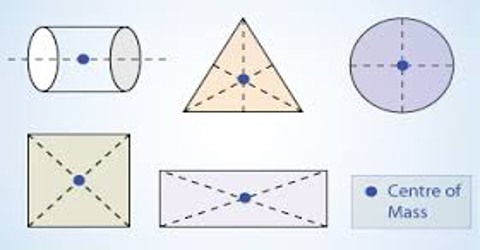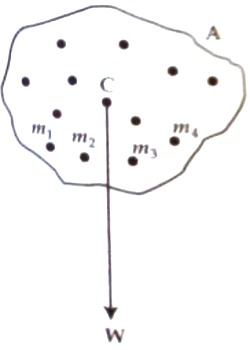Physics

# Centre of MassCentre of mass

We know, a body is composed of many small particles. If it is considered that all the masses of these small particles are concentrated at a point, then the resultant of all the parallel forces those are proportional to their masses are considered to be acting through that point that point is called the centre of mass of the body. The center of mass is a position defined relative to an object or system of objects. It is the average position of all the parts of the system, weighted according to their masses.Let A be an object. It is composed of many small particles. Let the masses of these particles be m1, m2, m3, … … mn etc. (figure). If it is considered that all the masses are concentrated at point C, then the resultant of the forces on those particles that are proportional to their respective masses is acting through that point C. This point is called the centre of mass.